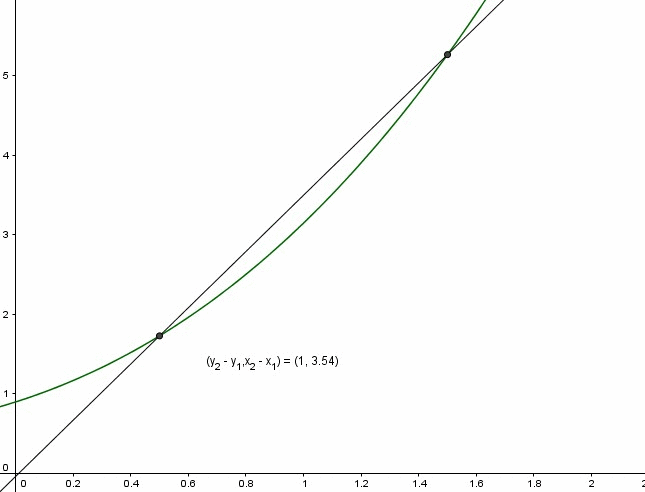# what makes some line to be a tangent line on to other Graph.

I learned tangent line as a concept associated with a circle. I thought to make a tangent line, it might have some specific point which is perpendicular to. as always did with circle did. But in the lesson of calculus, it doesn't seems any another point to associate. To draw tangent lines of some graph, how could we refer the slope of tangent line? Before we calculate?

I mean what makes some line to be a tangent line on the graph. How could be a tangent line with out any other point?

• The method we define tangent lines is via calculus. The only straight line goes through $(x,f(x))$ with slope $f'(x)$.
– Lab
May 14 at 6:05
• You should think of a line like $y = mx$ where $m$ (the slope) can be anything. This line always goes through the origin $(0, 0)$ and has different slopes. As you move this line (with $(0, 0)$ as its center) along a curve it will rotate continuously to stay tangent with the curve--these different orientations (or rotations) are the different slopes along the curve at certain points. May 14 at 6:07
• It doesn't make sense to say a line is perpendicular to a point, so I don't think you have the right idea for a tangent to a circle. A better (but still not perfect) way to think of a tangent to a curve at a point is as a line that, near that point, stays on one side of the curve. May 14 at 6:31
• Welcome to Math.SE! <> The title and question body don't quite match. Can someone provide the formal definition of the tangent line to a curve addresses the title question; the answers there (other than mine) may help. May 14 at 11:08

Here's an animation to see how the tangent line can be created using limits;